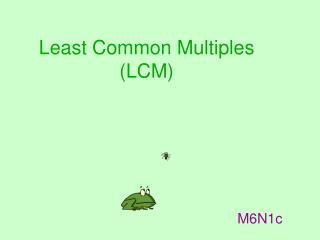DownloadDownload PresentationLeast Common Multiples (LCM)

# Least Common Multiples (LCM)

Download Presentation## Least Common Multiples (LCM)

- - - - - - - - - - - - - - - - - - - - - - - - - - - E N D - - - - - - - - - - - - - - - - - - - - - - - - - - -
##### Presentation Transcript

1. Least Common Multiple(LCM) • Smallest number that is a multiple of 2 or more numbers

2. Least Common Multiple Ladder Method

3. EXAMPLE Find the LCM of 6 and 10 STEP 1: Find the smallest prime number that goes into all of the given numbers. Continue until you have at least one prime number. STEP 2: Multiply all of the numbers on the side and on the bottom row. 2 ∙ 3 ∙ 5 = 30 2 6 10 3 5 LCM = 30

4. EXAMPLE Find the LCM of 6 and 12 2 6 12 3 6 3 1 2 2 ∙ 3 ∙ 1 ∙ 2 = 12 LCM = 12

5. EXAMPLE Find the LCM of 3 and 7 3 7 *Since both of the numbers are prime, simply multiply them together. 3 ∙ 7 = 21 LCM = 21

6. Find the LCM of 3, 9, and 12 3 9 12 3 4 3 ∙ 3 ∙ 4 = 36 YOUR TURN LCM = 36

7. Find the LCM of 3, 7, and 10 7 10 7 ∙ 10 = 70 LCM = 70 YOUR TURN

8. Find the LCM of 2 and 8 2 2 8 1 4 2 ∙ 1 ∙ 4 = 8 LCM = 8 YOUR TURN

9. Least Common Multiple(LCM) LISTING METHOD

10. EXAMPLE Find the LCM of 8 and 12 Step 1: List the multiples of each number Step 2: Circle the smallest number that is in all the lists. Step 3: Write the answer 8, 16, 24, 32 12, 24 LCM = 24

11. EXAMPLE Find the LCM of 3, 5, and 6 3, 6, 9, 12, 15, 18, 21, 24, 27, 30, 5, 10, 15, 20, 25, 30, 6, 12, 18, 24, 30, LCM = 30

12. Find the LCM of 3 and 5 3, 6, 9, 12, 15 5, 10, 15 YOUR TURN

13. Find the LCM of 6, and 9 6, 12, 18 9, 18 YOUR TURN

14. Problem Solving

15. Hot dogs come in packs of 8. Hot dog buns come in packs of 12. What is the least number of packs of each Shawn should buy to have enough to serve 24 people and have none left over? 3 packs of hot dogs and 2 packs of buns.

16. Debbie wants to invite 60 people to her party. Invitations come in packs of 12 and stamps come in sheets of 10. What is the least number of each she should buy to mail an invitation to each person and have no supplies left over? 5 packs of invitations and 6 sheets of stamps

17. You want to have an equal number of plastic cups and paper plates. What is the least number of packs of each you can buy? 3 packs of plates and 2 packs of cups

18. You want to have an equal number of noisemakers and balloons at your party. What is the least number of packs of each you can buy? 1 pack of balloons and 6 packs of noise makers.

19. Tony wants to make 36 party bags. Glitter pens come in packs of 6. Stickers come in sheets of 4, and balls come in packs of 3. What is the least number of each package he should buy to have 1 of each item in every party bag, and no supplies left over? 6 packs of pens, 9 sheets of stickers, and 12 packs of balls